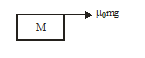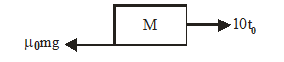Two block problem
Question

# A plank having mass M is placed on smooth horizontal surface. Block of mass m is placed on it coefficient of friction between block and plank is ${\mathrm{\mu }}_{0}+{\mathrm{kx}}^{2}$, where k is constant and x is relative displacement between block and plank. A force F is applied on block where F = at, where a = 10; t is in second. Find ${\mathrm{t}}_{0}$ when relative motion will occur between block and plankModerate
Solution

## Let at time ${\mathrm{t}}_{0}$ relative motion will occur${\mathrm{\mu }}_{0}\mathrm{mg}=\mathrm{Ma}\text{\hspace{0.17em}\hspace{0.17em}\hspace{0.17em}\hspace{0.17em}}......\left(1\right)$$10\text{\hspace{0.17em}}{\mathrm{t}}_{0}-{\mathrm{\mu }}_{0}\mathrm{mg}=\mathrm{ma}\text{\hspace{0.17em}\hspace{0.17em}\hspace{0.17em}}.......\left(2\right)$From (1) and (2) ; ${\mathrm{t}}_{0}={\mathrm{\mu }}_{0}\mathrm{m}+\frac{{\mathrm{\mu }}_{0}{\mathrm{m}}^{2}}{\mathrm{M}}$

Get Instant Solutions